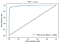# Predicting Pulsar Stars Using Neural Networks

Neutron stars are dense and hot objects, they provide unique opportunities to study the laws of physics in extreme conditions. Pulsars are highly magnetized neutron stars that rotate very fast and yield detectable periodic strong radio emissions.

Modern pulsar surveys produce large volumes of data, the process of manually labeling candidates is laborious and time consuming. Hence researchers are currently trying to study and come up with approaches for automatic identifying candidates.

The HTRU2 dataset describes a sample of pulsar candidates collected during the High Time Resolution Universe Survey. The dataset contains a total of 17 898 observations, where 1 639 are positive examples, and 16 259 are negative. For each candidate (observation) 8 continuous variables, and a
binary classification variable, are available:

1. the mean of the integrated profile;
2. the standard deviation of the integrated profile;
3. the excess kurtosis of the integrated profile;
4. the skewness of the integrated profile;
5. the mean of the DM-SNR curve;
6. the standard deviation of the DM-SNR curve;
7. the excess kurtosis of the DM-SNR curve;
8. the skewness of the DM-SNR curve;
9. and, the target class, where the values used are 1 for candidates
identified positively as pulsars, and 0 otherwise.

All the variables are available for every observation, Figure 1 illustrates a sample of the dataset.

The goal is to build a model that is able to identify a pulsar given a set of input features, we approach this as a classification problem. To implement the model we use a 2-layer neural network, illustrated in Figure 2, with a fully connected hidden network with 6 nodes using a RELU activation function, and output layer with a sigmoid activation function.

Next we fit the model to the dataset, using an Adam optimizer, and a binary cross entropy loss function.

The goal of the model created in previous sections is to correctly predict the identification of a pulsar given the defined features. Given enough training data the model should capture the inherent patterns of the data that identify a pulsar, generic enough that can be used to identify new cases, never seen by the model or training process. The evaluation of the model helps measuring the ability of the model to correctly generalize to unseen data.

Since the two classes of observations on the dataset are unbalanced, i.e. the number of positive observations (~ 10%) is much smaller than the negative ones (~ 90%), measuring accuracy is not a good approach to evaluate the model. To get a better grasp of the model performance we take a look at the ROC curve, a plot where the x-axis yields the number of false positive rate, and the y-axis yields the true positive rate, illustrated in Figure 3.Figure 3: Evaluating the model using a ROC curve, while predicting the classification on the test set.

An area of 1 under the line would represent a perfect classifier, i.e. all the observations were correctly predicted, an area of 0.5 represents a worthless classifier. In this case an area of 0.981 usually portrays a very good to excellent classifier.

The complete implementation of the model, including the evaluation and training stages discussed are available in a Kaggle kernel here, the dataset was originally published here.

## More from Nuno Ramos Carvalho

https://nunorc.github.io